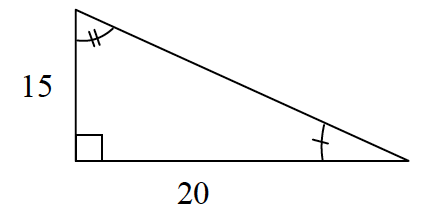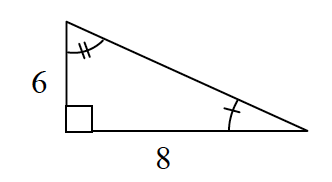### Home > GC > Chapter 3 > Lesson 3.1.2 > Problem3-18

3-18.

The ratios Casey wrote from the table in part (a) of problem 3-14 are common ratios between corresponding sides of the two shapes. That is, they are ratios between the matching sides of two shapes.

1. Look at the two similar shapes below. Which sides correspond? Write common ratios with the names of sides and lengths, just like Bernhard did.2. Find the hypotenuse of each triangle above. Is the ratio of the hypotenuses equal the ratios you found in part (a)?

The sides labeled $15$ and $6$ are corresponding, as are the sides labeled $20$ and $8$.

Refer to the Math Notes box in this section (3.1.2) if you need help writing common ratios.

Use the Pythagorean Theorem to solve for the hypotenuse.

Use this method to find the hypotenuse of the second triangle.
Is the ratio of the hypotenuses equal to the ratios you wrote in part (a)?

$15² + 20² = c²$

$625 = c²$

$\sqrt{625} = \sqrt{c^2}$

$c = 25$
(Note: the second solution $-25$ is rejected for this problem.)